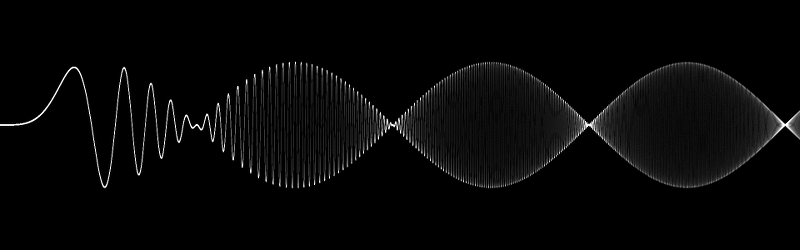PyCUDA Tutorial 英文原文

## 开始使用

``````import pycuda.driver as cuda
import pycuda.autoinit
from pycuda.compiler import SourceModule``````

## 转移数据

``````import numpy
a = numpy.random.randn(4,4)``````

``a = a.astype(numpy.float32)``

``a_gpu = cuda.mem_alloc(a.nbytes)``

``cuda.memcpy_htod(a_gpu, a)``

## 运行一个内核函数（kernel）

``````mod = SourceModule("""
__global__ void doublify(float *a)
{
a[idx] *= 2;
}
""")``````

``````func = mod.get_function("doublify")
func(a_gpu, block=(4,4,1))``````

``````a_doubled = numpy.empty_like(a)
cuda.memcpy_dtoh(a_doubled, a_gpu)
print (a_doubled)
print (a)``````

``````[[ 0.51360393  1.40589952  2.25009012  3.02563429]
[-0.75841576 -1.18757617  2.72269917  3.12156057]
[ 0.28826082 -2.92448163  1.21624792  2.86353827]
[ 1.57651746  0.63500965  2.21570683 -0.44537592]]
[[ 0.25680196  0.70294976  1.12504506  1.51281714]
[-0.37920788 -0.59378809  1.36134958  1.56078029]
[ 0.14413041 -1.46224082  0.60812396  1.43176913]
[ 0.78825873  0.31750482  1.10785341 -0.22268796]]``````

(本文的代码在PyCuda源代码目录下的examples/demo.py文件中。)

## 简化内存拷贝

PyCuda提供了pycuda.driver.In, pycuda.driver.Out, 以及pycuda.driver.InOut 这三个参数处理器（argument handlers），能用来简化内存和显存之间的数据拷贝。例如，咱们可以不去创建一个a_gpu，而是直接把a移动过去，下面的代码就可以实现：

``func(cuda.InOut(a), block=(4, 4, 1))``

## 有准备地调用函数

``````grid = (1, 1)
block = (4, 4, 1)
func.prepare("P")
func.prepared_call(grid, block, a_gpu)``````

## 抽象以降低复杂度

``````import pycuda.gpuarray as gpuarray
import pycuda.driver as cuda
import pycuda.autoinit
import numpy

a_gpu = gpuarray.to_gpu(numpy.random.randn(4,4).astype(numpy.float32))
a_doubled = (2*a_gpu).get()
print(a_doubled)
print(a_gpu)``````

## 结构体

（由Nicholas Tung提供，代码在examples/demo_struct.py文件中)

``````mod = SourceModule("""
struct DoubleOperation {
int datalen, __padding; // so 64-bit ptrs can be aligned
float *ptr;
};

__global__ void double_array(DoubleOperation *a) {
a = &a[blockIdx.x];
for (int idx = threadIdx.x; idx datalen; idx += blockDim.x) {
a->ptr[idx] *= 2;
}
}
""")``````

``````class DoubleOpStruct:
mem_size = 8 + numpy.intp(0).nbytes
def __init__(self, array, struct_arr_ptr):
self.data = cuda.to_device(array)
self.shape, self.dtype = array.shape, array.dtype
cuda.memcpy_htod(int(struct_arr_ptr), numpy.getbuffer(numpy.int32(array.size)))
cuda.memcpy_htod(int(struct_arr_ptr) + 8, numpy.getbuffer(numpy.intp(int(self.data))))
def __str__(self):
return str(cuda.from_device(self.data, self.shape, self.dtype))

struct_arr = cuda.mem_alloc(2 * DoubleOpStruct.mem_size)
do2_ptr = int(struct_arr) + DoubleOpStruct.mem_size

array1 = DoubleOpStruct(numpy.array([1, 2, 3], dtype=numpy.float32), struct_arr)
array2 = DoubleOpStruct(numpy.array([0, 4], dtype=numpy.float32), do2_ptr)
print("original arrays", array1, array2)``````

``````func = mod.get_function("double_array")
func(struct_arr, block = (32, 1, 1), grid=(2, 1))
print("doubled arrays", array1, array2)

func(numpy.intp(do2_ptr), block = (32, 1, 1), grid=(1, 1))
print("doubled second only", array1, array2, "\n")``````